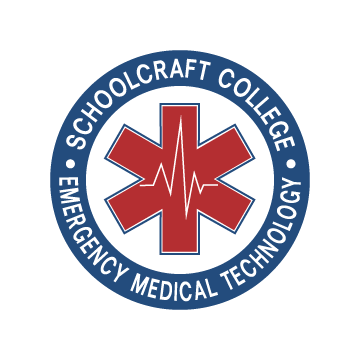# ECG Identification

20 Questions | Total Attempts: 64SettingsAs an emergency medical technician, one must have proper knowledge of how the heartbeat of a patient is through electrocardiography. The quiz below by the school craft college helps a student better gather information on whether the heart is working normally. Give the questions a try and see how well you will do as an EMT.

Related Topics
• 1.
• A.

Regular Sinus Rhythm

• B.

3rd Degree Heart Block

• C.

Sinus Rhythm with a 1st Degree Block

• D.

2nd Degree Type 2 2:1 AV Block

• 2.
• 3.
QRS-complex has its origin in
• A.

Repolarization of ventricles

• B.

Depolarization of atria

• C.

Depolarization of ventricles

• 4.
The delay of the contraction of ventricles after the contraction of atria is cause by the delay in
• A.

AV node

• B.

SA node

• C.

Septum

• 5.
T-wave has its origin in
• A.

Repolarization of ventricles

• B.

Repolarization of atria

• C.

Depolarization of ventricles

• 6.
• A.

Accelerated Juntional

• B.

Accelerated Idioventricular

• C.

Regular Sinus with 1st Degree AVB

• D.

Wandering Pacemaker

• 7.
The P-wave has its origin in
• A.

Repolarization of atria

• B.

Depolarization of atria

• C.

Depolarization of ventricles

• 8.
An ECG does not use the heart's electrical impulses/signals to determine whether a heart pattern is normal or abnormal?
• A.

True

• B.

False

• 9.
The right atrium receives oxygen rich blood.
• A.

True

• B.

False

• 10.
Which of the following is NOT one of the waves of the heart used by an ECG machine?
• A.

P

• B.

V

• C.

Q

• D.

T

• 11.
In a normal ECG, the heart should beat:
• A.

50-100 times per minute

• B.

60-100 times per minute

• C.

80-100 times per minute

• 12.
• A.

Multifocal Atrial Tachycardia

• B.

Atrial Tachycardia

• C.

Sinus Tachycardia

• D.

Supraventricular Tachycardia

• 13.
• A.

• B.

Sinus with PVC Bigeminy

• C.

• D.

Sinus with PAC Bigeminy

• 14.
The major intracellular ion is
• A.

Sodium

• B.

Potassium

• C.

Magnesium

• D.

Calcium

• 15.
The major extra cellular ion is
• A.

Sodium

• B.

Potassium

• C.

Magnesium

• D.

Calcium

• 16.
The heart rate is decreased to 40 bpm and stroke volume remains to 70 ml / beat. What is the cardiac output and whether the cardiac output is the same, increased or decreased?
• A.

3400ml / min cardiac output is decreased

• B.

2800 ml / min cardiac output is decreased

• C.

4800ml / min cardiac output remains the same

• D.

11400 ml / min cardiac output is increased

• 17.
A Normal Sinus Rhythm (NSR) represents: (choose the best answer)
• A.

Oxygenation impulses between the lungs and heart

• B.

Degree of strength of the contraction of heart muscle tissue

• C.

Electrical activity of the heart

• D.

Complete inactivity of the nares

• 18.
Which of the following wave sets and complexes represents sinus rhythm?
• A.

P wave followed by T wave then QRS complex

• B.

P wave followd by QRS complex then T wave

• C.

QRS complex followed by P wave then T wave

• D.

T wave followed by QRS complex the ventricular repolarization

• 19.
Variations in electrical impulses of the heart are collectively called:
• A.

Interval dysfunctions

• B.

Irregular spikes

• C.

Rate pattern impulses(rpi's)

• D.

Dysrhythmias

• 20.
Mr. Smith's heart rate is 120 beats per minute (BPM).  You would be correct in describing this rate as a:
• A.

• B.

Normal rate

• C.

Tachycardia

• D.

Heart block

• 21.
The part of the rhythm that indicates the time it takes for the sinoatrial node to fire and the atria to depolarize is known as the:
• A.

P wave

• B.

R wave

• C.

S wave

• D.

T wave